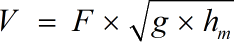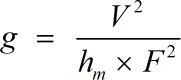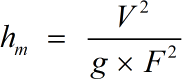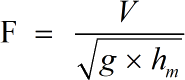Request a Tool

Froude Number Calculator

Froude number is defined as the ratio of a body's inertia to gravitational forces.

Flow Velocity
0

Formula• V = Flow Velocity
• g = Acceleration of Gravity
• hm = Mean Depth
• F = Froude Number

Defination / Uses

The dimensionless number that represents a ratio of a characteristic velocity to a gravitational wave velocity is known as the Froude Number. In simple terms, the Froude number is the ratio of inertia to gravitational forces. The symbol 'Fr' is used to represent it.

Output

Acceleration of Gravity
0

Formula• g = Acceleration of Gravity
• V = Flow Velocity
• hm = Mean Depth
• F = Froude Number

Defination / Uses

The dimensionless number that represents a ratio of a characteristic velocity to a gravitational wave velocity is known as the Froude Number. In simple terms, the Froude number is the ratio of inertia to gravitational forces. The symbol 'Fr' is used to represent it.

Mean Depth
0

Formula• hm = Mean Depth
• V = Flow Velocity
• g = Acceleration of Gravity
• F = Froude Number

Defination / Uses

The dimensionless number that represents a ratio of a characteristic velocity to a gravitational wave velocity is known as the Froude Number. In simple terms, the Froude number is the ratio of inertia to gravitational forces. The symbol 'Fr' is used to represent it.

Froude Number
0

Formula• F = Froude Number
• V = Flow Velocity
• g = Acceleration of Gravity
• hm = Mean Depth

Defination / Uses

The dimensionless number that represents a ratio of a characteristic velocity to a gravitational wave velocity is known as the Froude Number. In simple terms, the Froude number is the ratio of inertia to gravitational forces. The symbol 'Fr' is used to represent it.

How calculte the froude number?

There are only two basic steps.

• First select the option from dropdown. What you need to calculate.
• Then add the other values in their corresponding fields.

Thats it! You will get your answer in less than a second. Use upper given formula in case of manual calculation.

In hydrodynamics, the Froude Number is used to indicate how effectively a model functions in relation to a real system. The Froude Number Calculator can be used to calculate the Froude Number by entering the values for Flow Velocity (V), Gravity Acceleration (g), and Mean Depth (hm) and then applying the formula. The Froude Number can be calculated using our online Advanced Froude Number Calculator by entering the values for Flow Velocity (V), Acceleration of Gravity (g), and Mean Depth (hm) and applying the algorithm. For quick calculation use our weetools. No sign-up, registration OR captcha is required to use this tool.